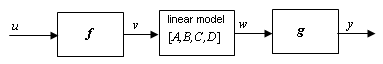idnlhw/linearize

Linearize Hammerstein-Wiener model

Syntax

SYS = linearize(NLSYS,U0)
SYS = linearize(NLSYS,U0,X0)

Description

SYS = linearize(NLSYS,U0) linearizes a Hammerstein-Wiener model around the equilibrium operating point. When using this syntax, equilibrium state values for the linearization are calculated automatically using U0.

SYS = linearize(NLSYS,U0,X0) linearizes the idnlhw model NLSYS around the operating point specified by the input U0 and state values X0. In this usage, X0 need not contain equilibrium state values. For more information about the definition of states for idnlhw models, see Definition of idnlhw States.

The output is a linear model that is the best linear approximation for inputs that vary in a small neighborhood of a constant input u(t) = U. The linearization is based on tangent linearization.

Input Arguments

• NLSYS: idnlhw model.

• U0: Matrix containing the constant input values for the model.

• X0: Operating point state values for the model.

Note

To estimate U0 and X0 from operating point specifications, use the findop command.

Output Arguments

• SYS is an idss model.

When the Control System Toolbox™ product is installed, SYS is an LTI object.

Algorithms

The idnlhw model structure represents a nonlinear system using a linear system connected in series with one or two static nonlinear systems. For example, you can use a static nonlinearity to simulate saturation or dead-zone behavior. The following figure shows the nonlinear system as a linear system that is modified by static input and output nonlinearities, where function f represents the input nonlinearity, g represents the output nonlinearity, and [A,B,C,D] represents a state-space parameterization of the linear model.The following equations govern the dynamics of an idnlhw model:

v(t) = f(u(t))

X(t+1) = AX(t)+Bv(t)

w(t) = CX(t)+Dv(t)

y(t) = g(w(t))

where

• u is the input signal

• v and w are intermediate signals (outputs of the input nonlinearity and linear model respectively)

• y is the model output

The linear approximation of the Hammerstein-Wiener model around an operating point (X*, u*) is as follows:

$\begin{array}{l}\Delta X\left(t+1\right)=A\Delta X\left(t\right)+B{f}_{u}\Delta u\left(t\right)\\ \Delta y\left(t\right)\approx {g}_{w}C\Delta X\left(t\right)+{g}_{w}D{f}_{u}\Delta u\left(t\right)\end{array}$

where

• $\Delta X\left(t\right)=X\left(t\right)-{X}^{*}\left(t\right)$

• $\Delta u\left(t\right)=u\left(t\right)-{u}^{*}\left(t\right)$

• $\Delta y\left(t\right)=y\left(t\right)-{y}^{*}\left(t\right)$

• ${{f}_{u}=\frac{\partial }{\partial u}f\left(u\right)|}_{u=u*}$

• ${{g}_{w}=\frac{\partial }{\partial w}g\left(w\right)|}_{w=w*}$

where y* is the output of the model corresponding to input u* and state vector X*, v* = f(u*), and w* is the response of the linear model for input v* and state X*.

Note

For linear approximations over larger input ranges, use linapp. For more information, see the linapp reference page.